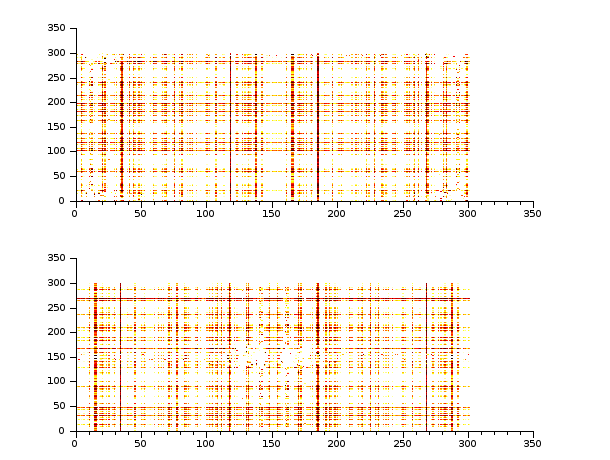Change language to:
Français - 日本語 - Português - Русский

See the recommended documentation of this function

Scilab help >> Signal Processing > fftshift

# fftshift

rearranges the fft output, moving the zero frequency to the center of the spectrum

### Calling Sequence

`y=fftshift(x [,job])`

### Arguments

x

real or complex vector or matrix.

y

real or complex vector or matrix.

job

integer, dimension selection, or string 'all'

### Description

if `x` results of an fft computation `y= fftshift(x)` or `y= fftshift(x,"all")` moves the zero frequency component to the center of the spectrum, which is sometimes a more convenient form.

If `x` is a vector of size n, y is the vector `x([n/2+1:n,1:n/2])`

If `x` is an `m` by `n` matrix `y` is the matrix `x([m/2+1:n,1:m/2],[n/2+1:n,1:n/2])`.

```[x11 x12]                  [x22 x21]
x=[       ]        gives   y=[       ]
[x21 x22]                  [x12 x11]```

`y= fftshift(x,n)` make the swap only along the `n`th dimension

### Examples

```//make a signal
t=0:0.1:1000;
x=3*sin(t)+8*sin(3*t)+0.5*sin(5*t)+3*rand(t);
//compute the fft
y=fft(x,-1);

//display
clf();
subplot(2,1,1);plot2d(abs(y))
subplot(2,1,2);plot2d(fftshift(abs(y)))``````//make a 2D image
t=0:0.1:30;
x=3*sin(t')*cos(2*t)+8*sin(3*t')*sin(5*t)+..
0.5*sin(5*t')*sin(5*t)+3*rand(t')*rand(t);
//compute the fft
y=fft(x,-1);

//display
clf();
xset('colormap',hotcolormap(256))
subplot(2,1,1);Matplot(abs(y))
subplot(2,1,2);Matplot(fftshift(abs(y)))```